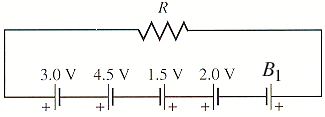# Circuits and resistors and batteries, oh my (a.k.a. I know nothing)

• rubytuesday

## Homework Statement## Homework Equations

I've been reading through the book but I don't really understand what I'm looking at. I know that V=I*R. I'm confused about the part between 1.5V and 2V--what does it mean that it's positive at both ends?

Adding up all the resistances will give me the emf (I think), and since I'm given B1 I'm supposed to be able to figure it out, but that positive-positive part throws me off.

Also, what happens if there were 2 or 3 in parallel instead of in series? There's a question later on that looks like the picture above but much uglier with billions of resistors in all different directions and it's really intimidating me since I can't even figure out this weeny problem.

## Homework Statement## Homework Equations

I've been reading through the book but I don't really understand what I'm looking at. I know that V=I*R. I'm confused about the part between 1.5V and 2V--what does it mean that it's positive at both ends?

Adding up all the resistances will give me the emf (I think), and since I'm given B1 I'm supposed to be able to figure it out, but that positive-positive part throws me off.

Also, what happens if there were 2 or 3 in parallel instead of in series? There's a question later on that looks like the picture above but much uglier with billions of resistors in all different directions and it's really intimidating me since I can't even figure out this weeny problem.

## The Attempt at a Solution

When you take a voltage loop then they merely add or subtract depending on whether they are adding or subtracting from positive voltage.

I would read that for instance as (8 - B1) V

Thanks! I did it the dumbed-down way (B1-2+1.5...) but you were right!

If resistors are in parallel, do you just divide the voltage among the resistors? Or is that completely off the wall? Sorry to bother you again.

Thanks! I did it the dumbed-down way (B1-2+1.5...) but you were right!

If resistors are in parallel, do you just divide the voltage among the resistors? Or is that completely off the wall? Sorry to bother you again.

Voltage is the same across all || Resistors.

It's current that splits.

If resistors are in series, then voltage divides.

Thank you!